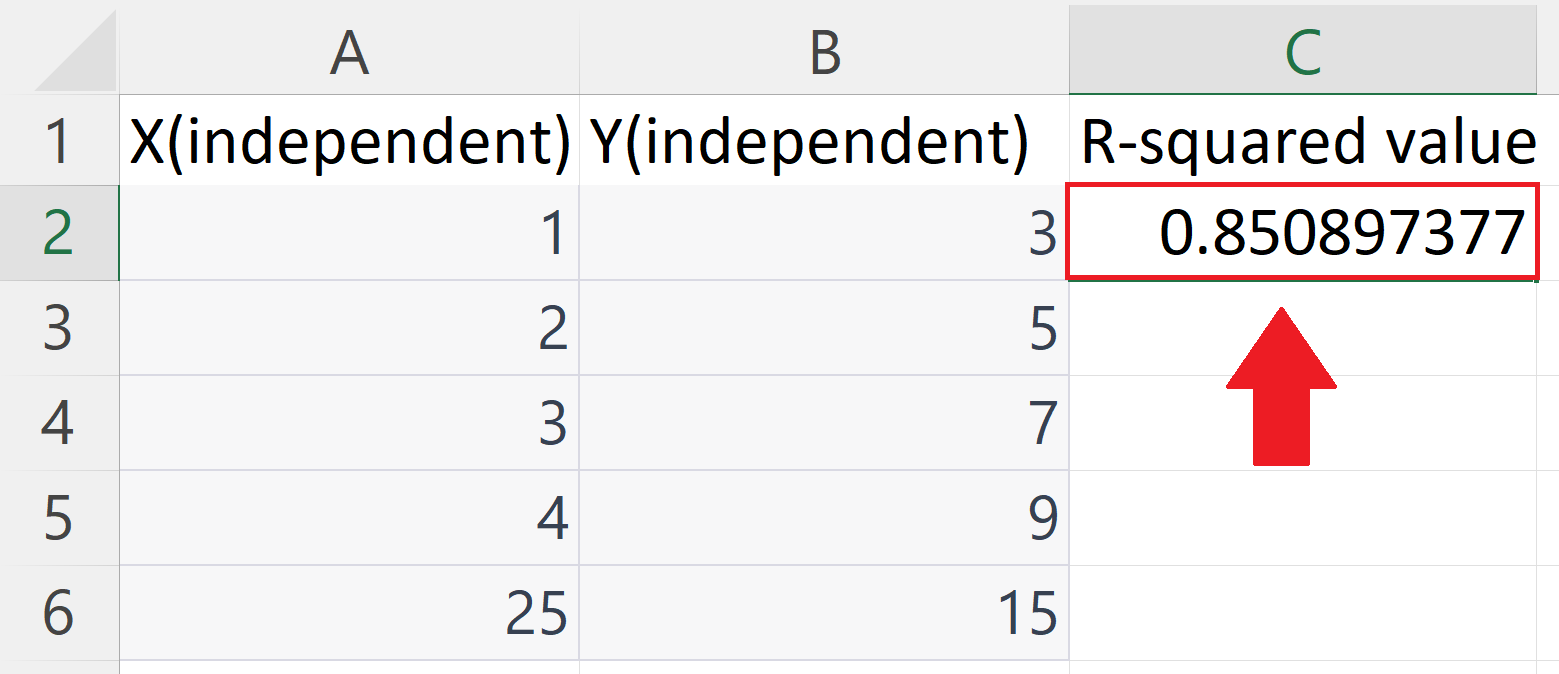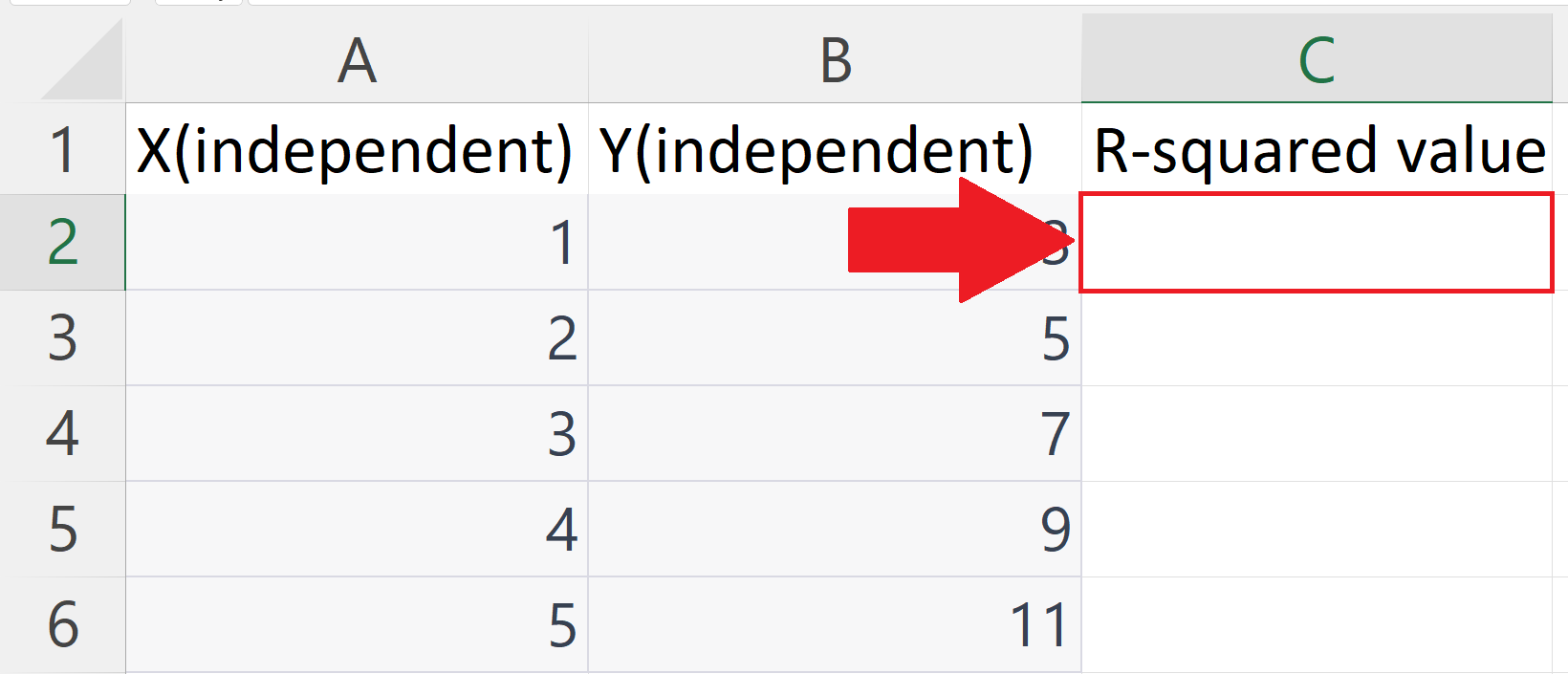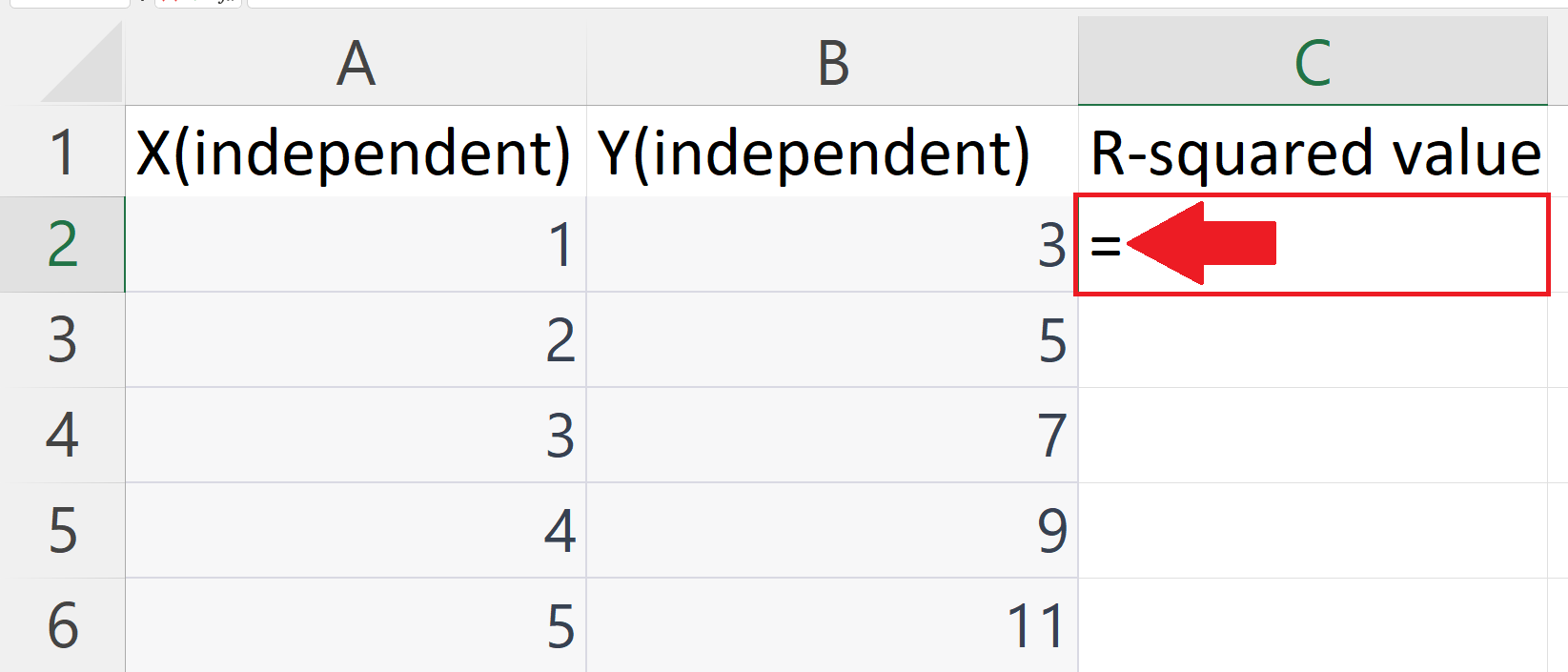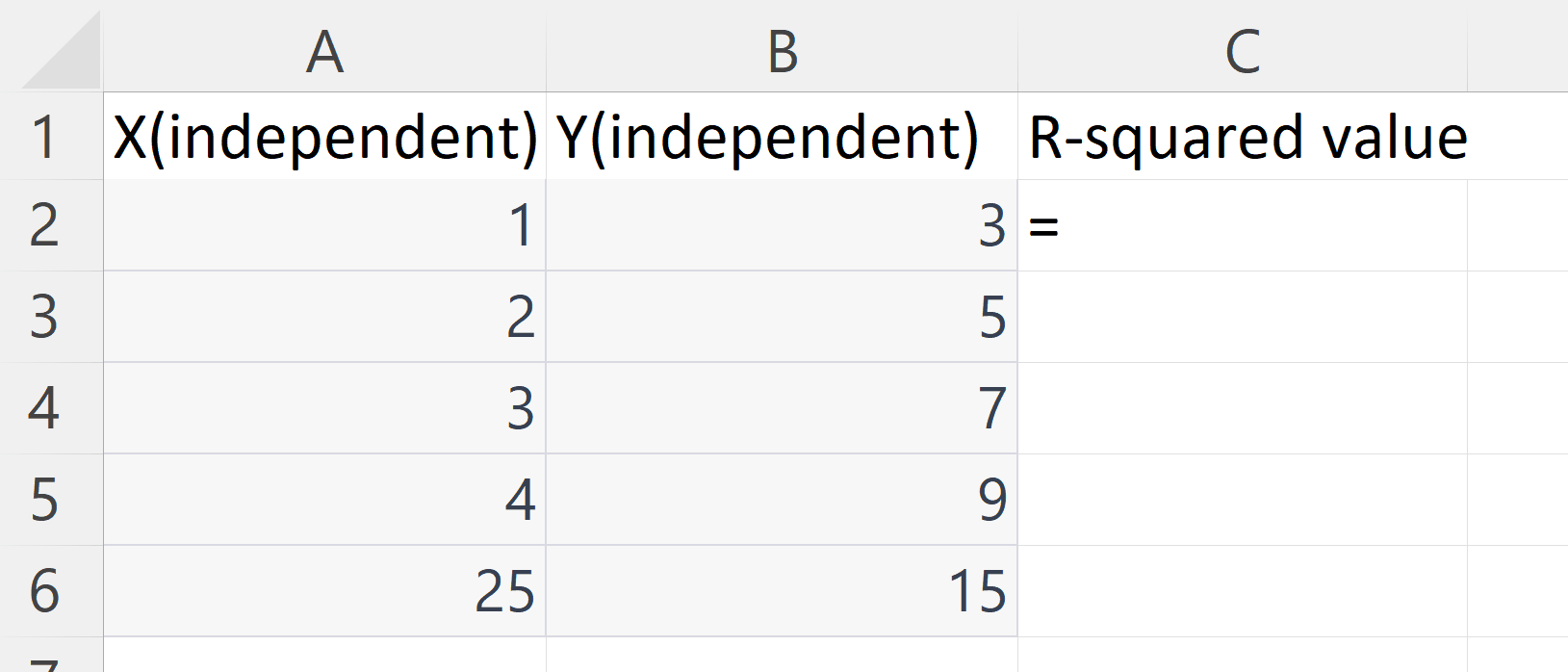# How to calculate R-squared value in Microsoft Excel

In this tutorial we will learn how to calculate R-squared value in Microsoft Excel. R-squared, also known as the coefficient of determination, is a statistical measure used in regression analysis to determine how well a model fits the data. In Microsoft Excel, R-squared is often used in conjunction with a linear regression analysis to evaluate the goodness of fit of a model. The R-squared value ranges from 0 to 1, where a value of 1 indicates that the model perfectly fits the data, and a value close to 0 indicates that the model does not fit the data well. In general, a high R-squared value indicates a strong correlation between the independent and dependent variables in the model, and a low R-squared value indicates a weak correlation.Microsoft Excel is a spreadsheet software program developed by Microsoft and is widely used for organizing, analyzing, and managing data. It is part of the Microsoft Office suite and is available for Windows and Mac platforms. Excel provides users with a vast array of features that make it easy to work with data, including the ability to create, edit, and manipulate data in cells; perform calculations with formulas and functions; create charts and graphs to visualize data; and perform data analysis with pivot tables and advanced filtering.

### Step 1 – Select a Blank Cell– Select a blank cell where you want to calculate the R-squared value.

### Step 2 – Place an Equals sign– Place an equals sign ( = ) in the blank cell.

### Step 3 – Use RSQ function– Use the RSQ function to calculate the R-squared value.
– The syntax of RSQ function is
RSQ(y,x)
– The first argument i.e. x is the range of cells containing known independent variables.
– The second argument i.e. y is the range of cells containing  known dependent variables.
– You may use the “Handle Select” and “Drag and Drop” to select the range of cells or enter the range manually.

### Step 4 – Press the Enter Key– Press the Enter key to get the R-square value.